# Average speed

Average speed is the ratio between the distance traveled by a car and the time interval in which it traveled that distance. The average speed is considered a scalar quantity , and is also known as speed , since it only takes into account the rate at which a certain space is covered.

## Average speed formula

The formula for average speed is the ratio of the distance traveled to the time interval, note: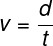v – average speed
d – distance traveled
t – time interval

When solving exercises that involve calculating the average speed, it is important that we pay attention to the units used. According to the international system of units , speed is expressed in the unit of meters per second (m/s), therefore, the distance traveled must be expressed in meters (m), and the time, in seconds (s). However, it is common to express speed in common units, such as kilometer per hour (km/h). If this is the case, you can convert m/s to km/h, or vice versa, using the following rule:

## Average speed and average speed

The main difference between the average speed and the average speed is that, in the latter, displacement is considered, which is a vector quantity determined by the difference between the final and initial positions of a mobile. The formula for the average speed is represented in the following figure, note: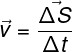v – average velocity
ΔS – displacement
Δt – time interval

The displacement of a mobile can be null in the case that it moves and returns to the initial position, however, the space covered by it will never be null .

## Average speed exercises

Question 1) Consider that Roberto, in his 2000 m walks to maintain his physical conditioning, develops an average speed of 5 km/h. The time taken to cover this distance is:

a) 12 min
b) 20 min
c) 24 min
d) 36 min
e) 40 min

Template: Letter C

Resolution:

First, let’s convert the distance traveled into km. Then we can calculate the time, check it out: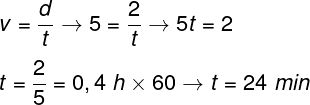At the end of the calculation, we multiply the time, which was in hours, by 60, to transform it into minutes.

Question 2) (UFPR) The use of GPS receivers is increasingly common in vehicles. The working principle of this instrument is based on the time interval of propagation of signals, through electromagnetic waves, from the satellites to the GPS receivers. Considering the propagation speed of the electromagnetic wave to be 300,000 km/s and that, at a given moment, one of the satellites is 30,000 km away from the receiver, what is the propagation time of the electromagnetic wave emitted by this GPS satellite until the receiver?

a) 10 s
b) 1 s
c) 0.1 s
d) 0.01 s
e) 1 ms

Template: Letter C

Resolution:

The exercise asks us to calculate the time in which the electromagnetic wave propagates. To do so, we will use the formula for average speed, check: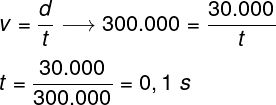Question 3) (UEA) With approximately 6500 km in length, the Amazon River competes with the Nile River for the title of the longest river on the planet. Assume that a drop of water traveling through the Amazon River has a speed equal to 18 km/h and that this speed remains constant throughout the journey. Under these conditions, the approximate time, in days, that this drop would take to travel the entire length of the river is:

a) 20
b) 35
c) 25
d) 30
e) 15

Template : Letter E

Resolution:

Let’s determine the number of hours required for the drop to cross the entire length of the Amazon River: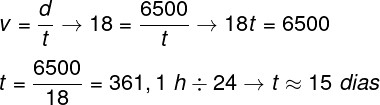Check Also
Close